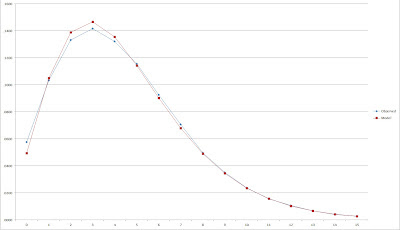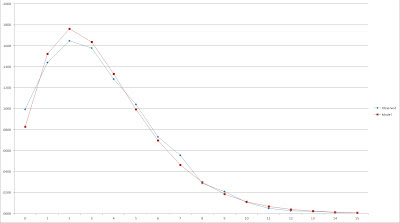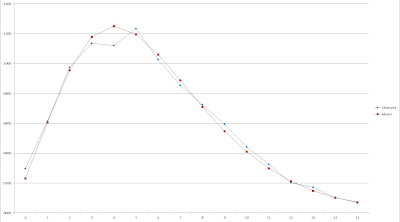## Monday, June 11, 2012

### On Run Distributions, pt. 2: Negative Binomial

There does exist a common discrete distribution that can be useful in modeling the per game run distribution. It doesn’t necessarily seem like it should work, since the questions that the distribution is typically employed to answer (at least in the sort of non-expert presentations most people are exposed to) doesn’t have an obvious connection with expected runs scored in baseball. Is it a perfect fit? Of course not; there are no perfect models anyway, but even granting that, it has flaws. Would it work in extreme circumstances? I don’t really know; over the next several articles I’ll take a stab at answering these questions, but obviously I have my limits as an analyst.

I did a couple of internet searches to try to see if there were any published papers or articles using the Negative Binomial distribution to model runs scored. There were a few that seemed to mention it, and there was an article about cricket scoring, but I didn’t see anything using it for the exact purpose I am. That does not mean none exist.

In any event, I know that I am not the first person to use the distribution to model runs per game, because I was sent an unpublished paper about it several years ago by Phil Melita. Melita sent it to me since he had cited Pythagenpat in the article, and we traded a couple emails about it. It was never published in By the Numbers, though, and I’m not sure why. In any event, Melita didn’t present his work in the same manner that I am here, but it was clearly along the same lines and I can take no credit for any originality here. I hadn’t thought about it in a few years, but as soon as I tried the negative binomial, I remembered Melita’s article.

To be honest, the negative binomial is not one of my favorite distributions. From my educational experiences, it is associated with two things in my mind: 1) the nice, simple Geometric distribution (a special case of negative binomial) run amuck and 2) Poisson-Gamma compound processes. It does have other obvious baseball uses, but it’s just not as intuitive to me as Poisson, Geometric, or Binomial.

The way the negative binomial distribution is usually presented masks its ability to deal with fractional parameters, hidden by the use of factorials and the aversion of everyone but mathematicians to gamma functions. And the problems to which the negative binomial is usually applied are along the lines of “What is the probability of 5 successes before the eighth failure?”, which further reduces the likelihood that people will consider it as a model for run scoring. However, the pmf for the negative binomial can be written thusly:

p(k) = (1 + B)^(-r) for k = 0
p(k) = (r)(r + 1)(r + 2)(r + 3)…(r + k - 1)*B^k/(k!*(1 + B)^(r + k)) for k >=1

where r and B are the parameters of the distribution and k is the point at which we are calculating the probability.

Of course, in order to actually use this or any other distribution, we need to be able to define the parameters. Everything that follows in this series of posts needs to be prefaced by this caveat--I am not a statistician or a probability expert. Compared to the average person, I have a lot of knowledge through my own curiosity and my studies/profession. Compared to the average person who dabbles in sabermetrics, though, my knowledge doesn’t stand out, and obviously I am a kindergartner compared to the experts in these fields.

So here I will take the easy step of fitting the parameters of this distribution using the method of moments. There may well be a better, more precise method out there (maximum likelihood estimation always comes to mind, but is a nightmare for negative binomial). In any event, the most important simple properties of any distribution are its mean and variance. For negative binomial, these are computed as follows:

mean (u) = r*B
variance(v) = r*B*(1 + B)

The method of moments uses the first two central moments (mean and variance to estimate the parameters). If we take the ratio of v to u, we can estimate B:

v/u = r*B*(1 + B)/(r*B) = 1 + B
Ergo B =v/u - 1 and r = u/B = u^2/(v - u).

For 1981-96, the average runs scored per game was 4.4558 with a variance of 9.3603, which leads to an estimate that B = 9.3603/4.4558 - 1 = 1.1007 and r = 4.4558/1.1007 = 4.0480.

Given those parameters, here are the estimates for the first few run totals:

p(0 runs) = (1 + B)^(-r) = (1 + 1.1007)^(-4.048) = .0496
p(1 run) = r*B^k/(k!*(1 + B)^(r + k)) = 4.048*1.1007/(1!*(1 + 1.1007)^(4.408 + 1)) = .1051
p(2 runs) = r*(r + 1)*B^k/(k!*(1 + B)^(r + k)) = 4.408*(4.408 + 1)*1.1007^2/(2!*(1 + 1.1007)^(4.408 + 2)) = .1390
p(3 runs) = r*(r + 1)*(r + 2)*B^k/(k!*(1 + B)^(r + k)) = 4.408*(4.408 + 1)*(4.408 + 2)*1.1007^3/(3!*(1 + 1.1007)^(4.408 + 3)) = .1468

The r*(r + 1)*(r + 2)*(r + 3)*(r + 4)*... formula gets annoying, but with a spreadsheet it is easy to implement. Here is the complete runout (to 16 runs), along with a graph comparing the estimate probabilities to the observed frequencies:When I look at that graph, I see a pretty decent fit, one that makes me believe that the negative binomial distribution may have some usefulness in estimating run distributions. Is it perfect? No; as you can see, it is noticeably low on shutouts (an issue that I will go into detail about in the next post), and it’s too high for some of the other common scores (2-4). Using the X^2 test statistic described in the previous post, this scores a 176, which is easily better than the other crude models I considered.

Of course, I’ve only dealt with scoring at normal levels. In order to estimate run distributions for other scoring levels, we’ll have to change the parameters. The mean number of runs per game is always known, but the variance is a quantity that we can only know if we have prior knowledge of the empirical run distribution. Since both r and B are dependent on both the mean and variance, they will be constantly changing when the mean and variance of the distribution we are fitting to changes.

Before worrying about how to tackle that problem, we first need to make sure it would be worth attempting to adapt to non-average situations. I will consider the top and bottom 5% of teams by R/G for the data in Davenport’s spreadsheet--the 25 lowest and highest scoring teams of 1981-2006. The bottom 25 teams averaged 3.49 R/G with a variance of 6.6206, which produces estimates of B = .897 and r = 3.891. The results:This doesn’t look as nice, but it still appears as if the model does a decent job. Remember that the sample size is not that large (25 teams is a smaller sample than a full major league season), so there’s the possibility of some random fluctuation. Again, the point here is not to perform a rigorous test, but rather to make sure the model isn’t completely out in left field.

The 25 highest scoring teams averaged 5.7 R/G with a variance of 12.4 for B = 1.175 and r = 4.849:Not bad. Next time, I’ll discuss a workaround for the underestimation at zero runs (albeit one that introduces a new stumbling block).

#### 3 comments:

1.The negative binomial distribution gets in statistical in negative binomial regression. It's a type of generalized linear model for count data where you have y=exp(mx + b). In NB regression the variance is assumed to NB distributed. Originally this kind of model would have been estimated with a normal distribution. The problem with the normal distribution is that the variance is assumed constant. In count data, large predicted values are expected to have larger variances. Poisson regression was a big advance for count data because it allowed larger predicted values to have larger variances. With a Poisson distribution, the variance is equal to the mean. In many data sets the variance is greater than the mean (very rarely it's smaller). Variance that is much larger than the mean is called overdispersion and this is where NB regression comes in. NB regression allows the variance to grow quadratically. There are other variance models, including quasi-Poisson which let's the variance increase linearly with the predicted value.
The reason the variance is so important, is that if you get it wrong, then the standard errors of the coefficients, f values, t values, and chi-squares can all be wrong.

Alan

2.The classic definition of the negative binomial seems like it corresponds to the question "how many times does the batting team get on base before accumulating three outs?" Which has a notable difference from runs scored: essentially only the 4th+ of these successes are scoring.

Now I'm surprised the fit is as good as it is. This might explain why the model doesn't have enough shutouts, but it seems like it would overdo it.

Does any of this work out under more rigor?

1.Eli,

I don't have the requisite knowledge to answer the question with statistical rigor, but what I can tell you (some of which I posted in parts 3-7 of this series and some of which I need to write up still) is:

1. When a correction for P(0 runs) is applied, the model appears to be reasonably accurate at estimating the distributions for major league teams with normal levels of run scoring

2. The results are consistent with those you would get from using Tango's runs per inning distribution (Tango Distribution) to estimate a runs per game distribution.

3. The estimates of W% that one can calculate by estimating a teams distribution of runs scored and runs allowed (i.e. then P(win) = P(score >0)*P(allow 0) + P(score >1)*P(allow <=1) + ...) are very similar to those found using other run/win converters (such as Pythagorean).

Comments are moderated, so there will be a lag between your post and it actually appearing. I reserve the right to reject any comment for any reason.